## 使用scikit-learn进行线性回归分析

Posted on May 9, 2018

# scikit-learn介绍

scikit-learn是Python语言的机器学习软件库。其官网地址在这里：scikit-learn

scikit-learn有如下特点：

• 它包含了简单高效的数据挖掘和数据分析工具
• 可供所有人使用，并可在各种环境下使用
• 基于NumPy，SciPy和matplotlib
• 基于BSD开源，因此开源项目和商业项目均可以使用

# 机器学习简介

• 监督学习（Supervised Learning）：数据包含了我们将要预测的附加属性。监督学习又可以分为：
• 分类（Classification）：样本属于两个或多个类别，我们希望从已标记的数据中学习如何预测未标记数据的类别。分类问题的一个例子是手写数字识别的例子，其中的目标是将每个输入向量分配给有限数量的离散类别之一。
• 回归（Regression）：如果预测的结果由一个或多个连续变量组成，则该任务称为回归。回归问题的一个例子是预测某个动物的长度与年龄和体重的关系。
• 无监督学习（Unsupervised Learning）：其中训练数据由一组没有任何相应目标值的输入向量x组成。这些问题的目标可能是发现数据中类似例子的集合，这称为集群（clustering），或确定输入空间内的数据分布，这称为密度估计（density estimation），或者从高维度投影数据将空间降低到二维或三维。

# 实验环境

• Apple OS X 10.13
• Python 3.6.3
• scikit-learn 0.19.1
• matplotlib 2.1.1
• numpy 1.13.3

# 实验描述

import pandas as pd


# 了解数据

print("Describe Data:")
print(input_data.describe())

print("\nFirst 10 rows:")


describe()是pandas提供的API，它会输出对于数据整体的统计描述信息，像下面这样：

Describe Data:
longitude      latitude  housing_median_age   total_rooms  \
count  20640.000000  20640.000000        20640.000000  20640.000000
mean    -119.569704     35.631861           28.639486   2635.763081
std        2.003532      2.135952           12.585558   2181.615252
min     -124.350000     32.540000            1.000000      2.000000
25%     -121.800000     33.930000           18.000000   1447.750000
50%     -118.490000     34.260000           29.000000   2127.000000
75%     -118.010000     37.710000           37.000000   3148.000000
max     -114.310000     41.950000           52.000000  39320.000000

total_bedrooms    population    households  median_income  \
count    20433.000000  20640.000000  20640.000000   20640.000000
mean       537.870553   1425.476744    499.539680       3.870671
std        421.385070   1132.462122    382.329753       1.899822
min          1.000000      3.000000      1.000000       0.499900
25%        296.000000    787.000000    280.000000       2.563400
50%        435.000000   1166.000000    409.000000       3.534800
75%        647.000000   1725.000000    605.000000       4.743250
max       6445.000000  35682.000000   6082.000000      15.000100

median_house_value
count        20640.000000
mean        206855.816909
std         115395.615874
min          14999.000000
25%         119600.000000
50%         179700.000000
75%         264725.000000
max         500001.000000


input_data.head(10)输出了数据的前10行。

First 10 rows:
longitude  latitude  housing_median_age  total_rooms  total_bedrooms  \
0    -122.23     37.88                41.0        880.0           129.0
1    -122.22     37.86                21.0       7099.0          1106.0
2    -122.24     37.85                52.0       1467.0           190.0
3    -122.25     37.85                52.0       1274.0           235.0
4    -122.25     37.85                52.0       1627.0           280.0
5    -122.25     37.85                52.0        919.0           213.0
6    -122.25     37.84                52.0       2535.0           489.0
7    -122.25     37.84                52.0       3104.0           687.0
8    -122.26     37.84                42.0       2555.0           665.0
9    -122.25     37.84                52.0       3549.0           707.0

population  households  median_income  median_house_value ocean_proximity
0       322.0       126.0         8.3252            452600.0        NEAR BAY
1      2401.0      1138.0         8.3014            358500.0        NEAR BAY
2       496.0       177.0         7.2574            352100.0        NEAR BAY
3       558.0       219.0         5.6431            341300.0        NEAR BAY
4       565.0       259.0         3.8462            342200.0        NEAR BAY
5       413.0       193.0         4.0368            269700.0        NEAR BAY
6      1094.0       514.0         3.6591            299200.0        NEAR BAY
7      1157.0       647.0         3.1200            241400.0        NEAR BAY
8      1206.0       595.0         2.0804            226700.0        NEAR BAY
9      1551.0       714.0         3.6912            261100.0        NEAR BAY


• 这组数据总计包含了20640条数据
• 数据分为longitude，latitude，housing_median_age，total_rooms，total_bedrooms，population，households，median_income，median_house_value，ocean_proximity一共10列
• 有些数据缺少total_bedrooms列的信息，因此其总共的有效数据只有20433条
• ocean_proximity的数据是字符串类型的，而其他列都是数字类型的
• median_house_value代表了房子的价值，这就是我们希望预测的数据

input_data.hist(bins=100, figsize=(20, 12))
plt.show()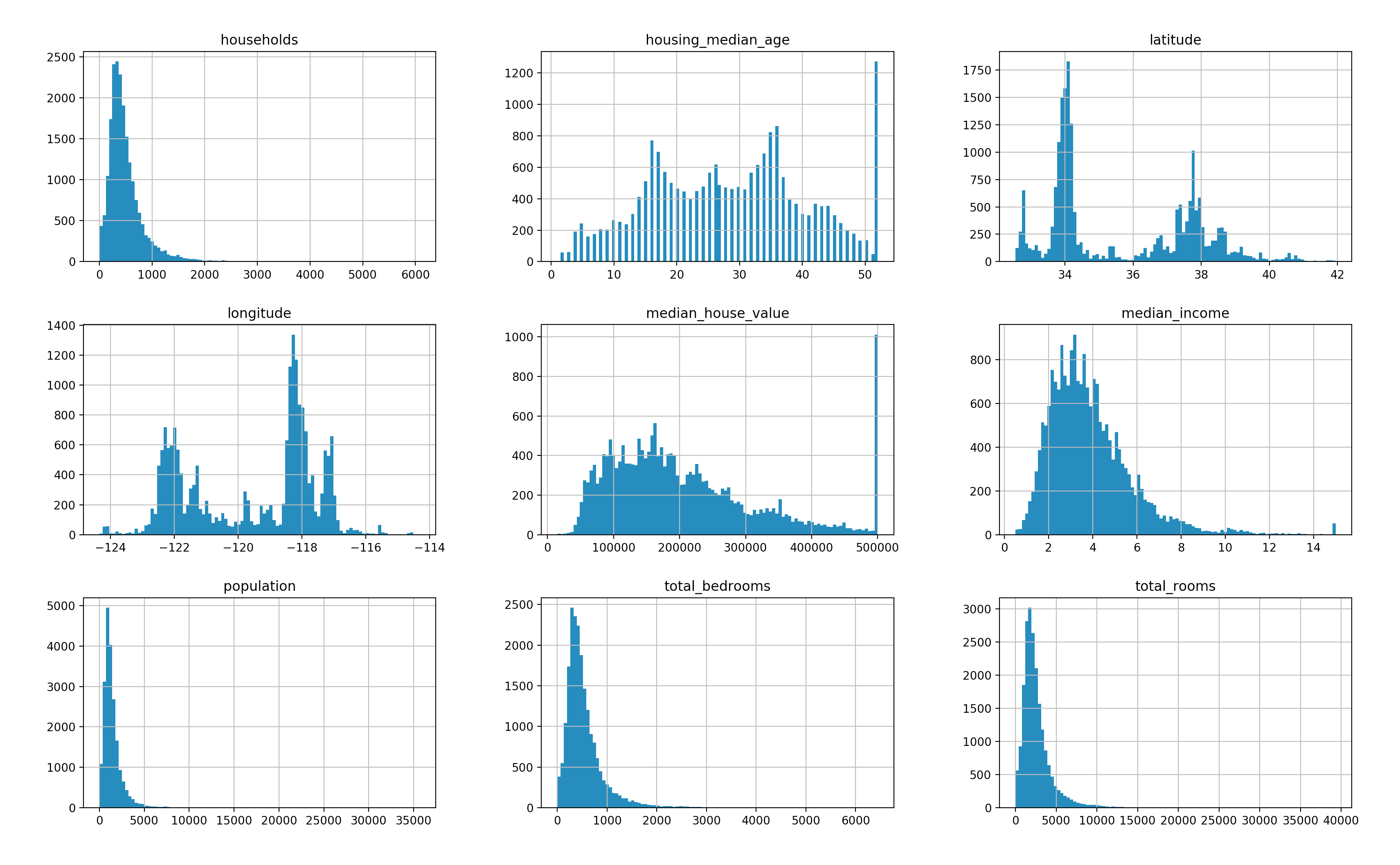input_data.plot(kind="scatter", x="longitude", y="latitude", alpha=0.1)
plt.show()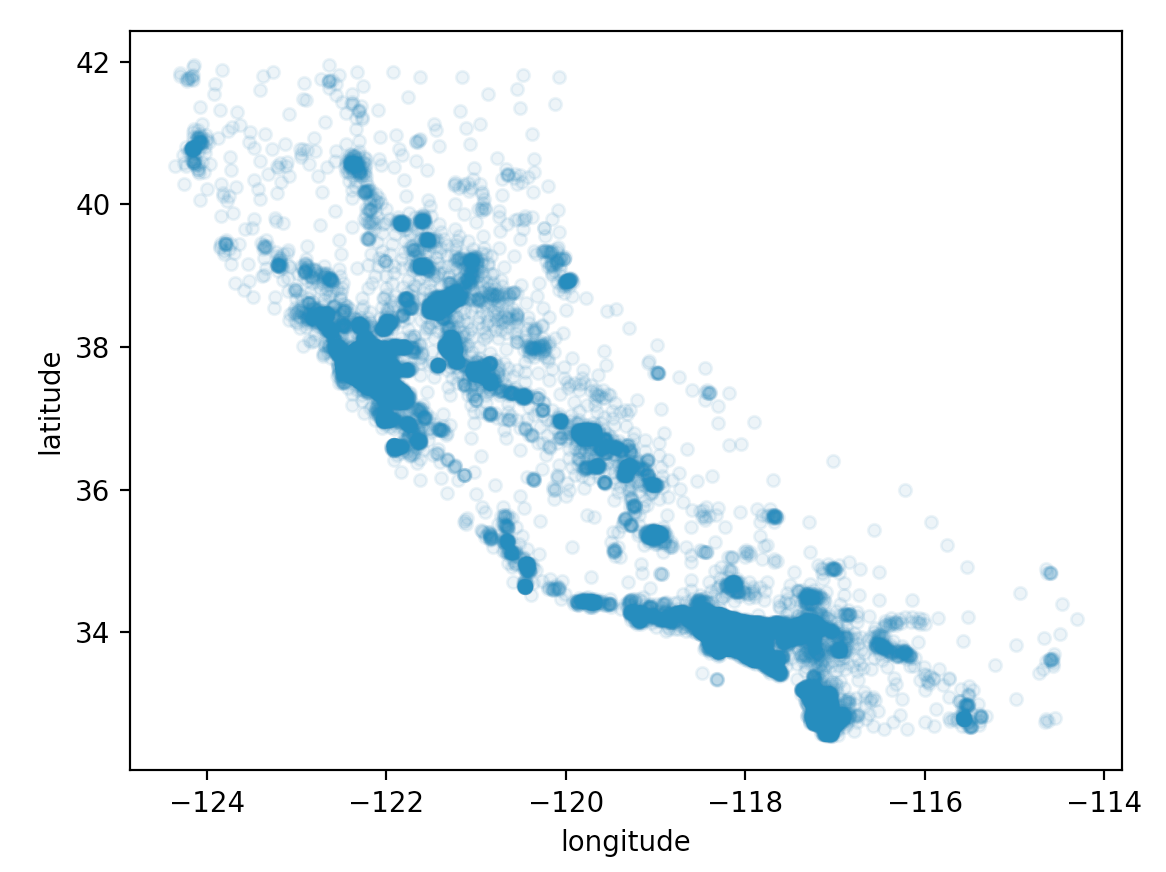# 预处理

## fit和transform

scikit-learn提供了一系列的转换器（Transformer）。它们用来可以清理，减少，展开和生成特征表示。这些类会具有下面三个方法：

• fit方法：该方法从训练集中学习模型参数（例如，用于归一化的均值和标准偏差）。
• transform方法：该方法对于数据进行模型变换。
• fit_transform方法：是前面两个方法的组合，它更加方便和高效。

sklearn.preprocessing中包含了一系列的工具进行数据的预处理。

## 处理字符串

from sklearn.preprocessing import LabelEncoder

encoder = LabelEncoder()
data["ocean_proximity"] = encoder.fit_transform(data["ocean_proximity"])


        ocean_proximity
count      20640.000000
mean           1.165843
std            1.420662
min            0.000000
25%            0.000000
50%            1.000000
75%            1.000000
max            4.000000


## 处理无效值

sklearn.preprocessing.Imputer 就是用来进行数值填充的。它提供了下面三种策略：

• “mean”：通过有效数值的平均值来填充
• “median”：通过有效数值的中位数来填充
• “most_frequent”：通过有效数值中出现频度最高的值来填充

import numpy as np

data = [1, 2, 3, 4, 5, 6, 7, 8, 95, 96, 97, 98, 100]

print("mean: {}".format(np.mean(data)))
print("median: {}".format(np.median(data)))


from sklearn.preprocessing import Imputer
imputer = Imputer(strategy="median")
X = imputer.fit_transform(data)
input_data = pd.DataFrame(X, columns=data.columns)


       total_bedrooms
count    20640.000000
mean       536.838857
std        419.391878
min          1.000000
25%        297.000000
50%        435.000000
75%        643.250000
max       6445.000000


## 特性缩放

MinMaxScaler用来通过指定数据的范围来进行数据的缩放。例如：

scalar = MinMaxScaler(feature_range=(0, 100), copy=False)
scalar.fit_transform(data)


plt.subplot(2, 1, 1)
plt.scatter(x=origin["longitude"], y=origin["latitude"],
c=origin["median_house_value"], cmap="viridis", alpha=0.1)
plt.subplot(2, 1, 2)
plt.scatter(x=scaled["longitude"], y=scaled["latitude"],
c=origin["median_house_value"], cmap="viridis", alpha=0.1)
plt.show()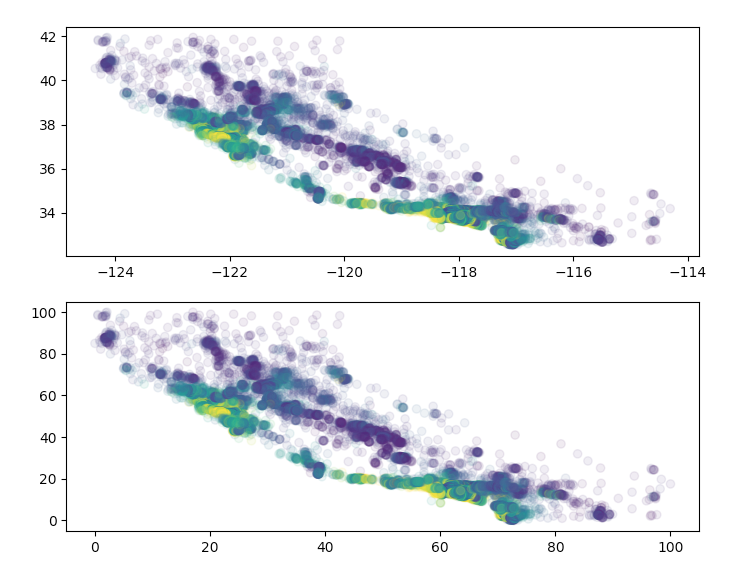# 数据划分

## permutation

permutation是numpy提供的接口。它将指定范围内的整数打乱以生成随机数。下面是一段代码示例：

import numpy as np

data = np.arange(0, 100)
np.random.seed(59)
print(data[np.random.permutation(100)[90:]])


[64 67  0 57 53 79 23 77 44 49]


## train_test_split

sklearn也提供了类似的功能。这个函数就是train_test_split。代码示例如下：

import numpy as np
from sklearn.model_selection import train_test_split

data = np.arange(0, 100)
train_set, test_set = train_test_split(data, test_size=0.1, random_state=59)
print("test_set: \n {} \n".format(test_set))


test_set:
[38 46 24 87 30 85 16 96 18 99]


train_set, test_set = train_test_split(input_data,
test_size=0.1, random_state=59)
show_data_summary(test_set)


Describe Data:
longitude     latitude  housing_median_age  total_rooms  \
count  2064.000000  2064.000000         2064.000000  2064.000000
mean     48.415061    32.276916           53.952918     6.692606
std      19.640685    22.608316           24.649983     5.817431
min       0.896414     0.000000            1.960784     0.022890
25%      27.863546    14.665250           33.333333     3.638919
50%      58.764940    17.959617           54.901961     5.348695
75%      63.346614    54.835282           70.588235     7.932118
max      96.613546    98.618491          100.000000    82.977262

total_bedrooms   population   households  median_income  \
count     2064.000000  2064.000000  2064.000000    2064.000000
mean         8.309936     4.013810     8.192199      22.725069
std          6.794665     3.581933     6.626166      12.800111
min          0.062073     0.028028     0.049334       0.000000
25%          4.527467     2.149724     4.534616      13.727052
50%          6.719429     3.188150     6.610755      20.242479
75%          9.970515     4.904846     9.965466      28.686501
max        100.000000    80.055495   100.000000     100.000000

median_house_value  ocean_proximity
count         2064.000000      2064.000000
mean            38.343739        28.234012
std             23.628904        34.874180
min              1.546592         0.000000
25%             20.350638         0.000000
50%             32.412444        25.000000
75%             49.432992        25.000000
max            100.000000       100.000000

First 10 rows:
longitude   latitude  housing_median_age  total_rooms  total_bedrooms  \
9878   24.800797  43.464400           70.588235     0.854570        1.675978
4356   59.661355  16.471838           72.549020     5.483494        9.016139
1434   23.107570  57.810840           84.313725     3.184292        3.895096
6422   63.247012  16.896918           45.098039     6.566967        8.054004
1624   22.011952  56.323061           45.098039     5.414823        5.307263
1423   22.908367  58.023379           29.411765     2.754464        3.351955
4853   60.258964  15.834219           70.588235     7.068010       11.871508
16893  19.621514  53.560043          100.000000     4.328806        3.491620
4232   60.258964  16.578108           70.588235    13.487461       30.710739
19083  18.625498  61.424017           80.392157     5.351239        8.255742

population  households  median_income  median_house_value  \
9878     0.639031    1.628022      14.009462           19.237240
4356     2.965330    9.472126      16.995628           70.164865
1434     1.387371    3.552047      20.366616           27.608340
6422     5.229967    8.255221      19.201114           31.340283
1624     2.441212    6.117415      35.413305           70.226721
1423     0.989378    3.798717      12.732928           12.371289
4853     7.239553   11.971715       9.355043           35.567070
16893    1.872250    3.979609      54.968207          100.000000
4232    10.908377   30.800855      10.808816           55.319566
19083    3.189551    7.301431      18.020441           27.690814

ocean_proximity
9878               0.0
4356               0.0
1434              75.0
6422              25.0
1624              75.0
1423              75.0
4853               0.0
16893            100.0
4232               0.0
19083              0.0
....


# 线性模型（linear model）

• 1999年，3800
• 2000年，3900
• 2001年，4000
• 2002年，4200
• 2003年，4500
• 2004年，5500
• 2005年，6500
• 2006年，7000
• 2007年，8000
• 2008年，8200
• 2009年，10000
• 2010年，14000
• 2011年，13850
• 2012年，13000
• 2013年，16000
• 2014年，18500

# house_price.py

import matplotlib.pyplot as plt
import numpy as np
import pandas as pd

data = pd.DataFrame({
"Year": [1999,2000,2001,2002,2003,2004,2005,2006,
2007,2008,2009,2010,2011,2012,2013,2014],
"Price": [3800,3900,4000,4200,4500,5500,6500,7000,
8000,8200,10000,14000,13850,13000,16000,18500]})
data.plot(kind="scatter", x="Year", y="Price", c="B", s=100)
plt.show()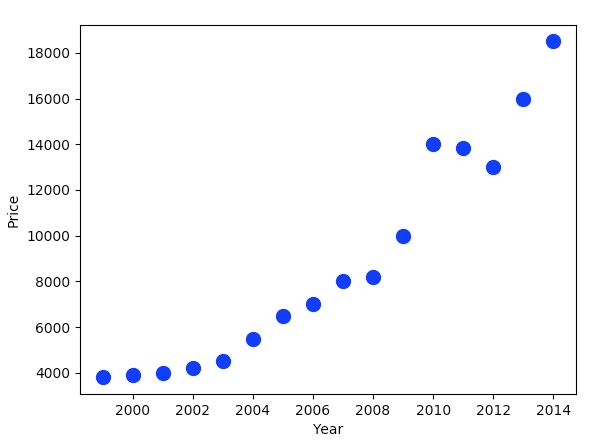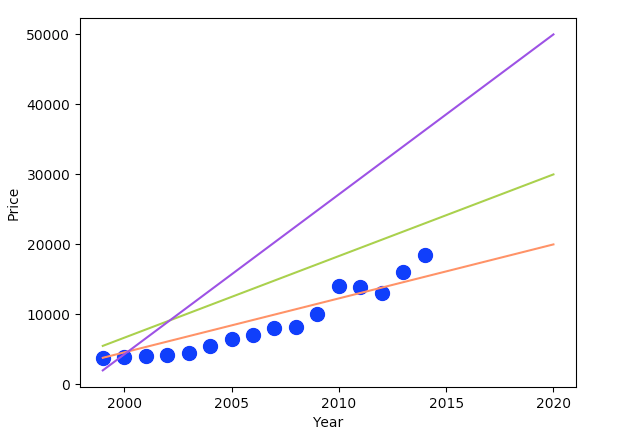$f(x) = wx + b$

$f(x) = w_{1}x_{1} + w_{2}x_{2} + ... + w_{n}x_{n} + b$

$f(x) = w^Tx + b$

def split_house_value(data):
value = data["median_house_value"].copy()
return data.drop(["median_house_value"], axis=1), value


train_set, test_set = train_test_split(input_data,
test_size=0.1, random_state=59)
train_data, train_value = split_house_value(train_set)
test_data, test_value = split_house_value(test_set)


from sklearn.linear_model import LinearRegression

linear_reg = LinearRegression()
linear_reg.fit(train_data, train_value)


predict_value = linear_reg.predict(test_data)


def show_predict_result(test_data, test_value, predict_value):
ax = plt.subplot(221)
plt.scatter(x=test_data["longitude"], y=test_data["latitude"],
s=test_value, c="dodgerblue", alpha=0.5)
plt.subplot(222)
plt.hist(test_value, color="dodgerblue")

plt.subplot(223)
plt.scatter(x=test_data["longitude"], y=test_data["latitude"],
s=predict_value, c="lightseagreen", alpha=0.5)
plt.subplot(224)
plt.hist(predict_value, color="lightseagreen")

plt.show()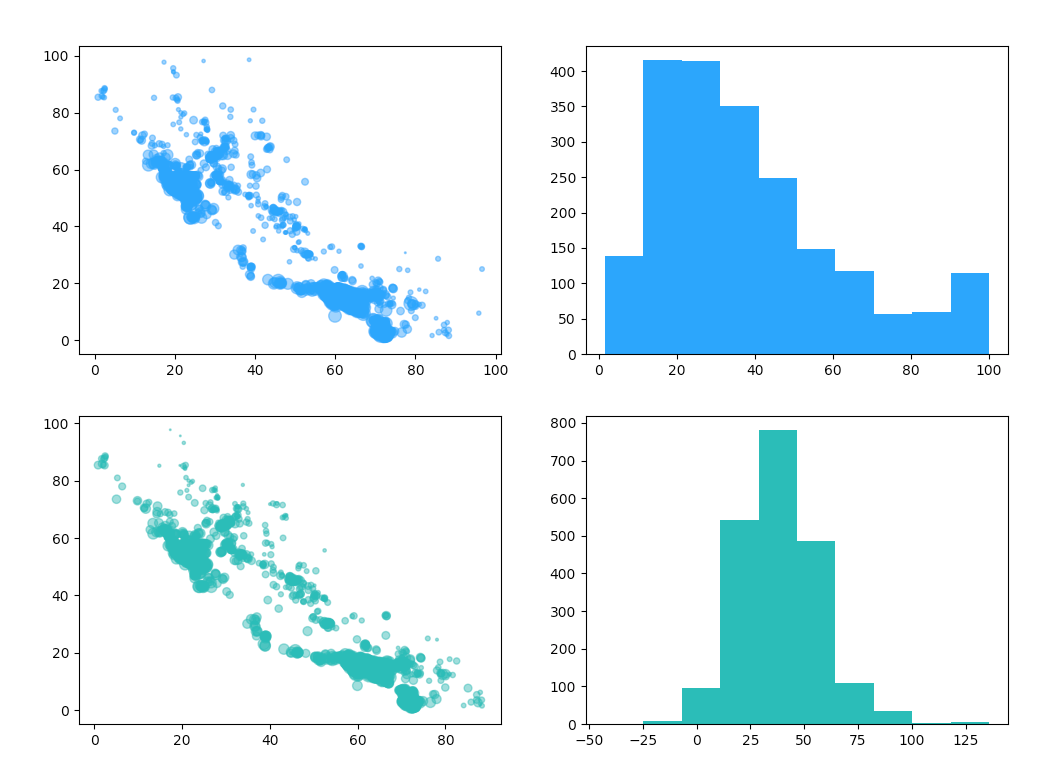# 性能度量

## 均方误差

$E(f) = \frac{1}{m}\sum_{i=1}^{m}(f(x_{i})-y_{i})^2$

from sklearn.metrics import mean_squared_error

mse = mean_squared_error(test_value, predict_value)
print("Diff: {}".format(np.sqrt(mse)))


Diff: 14.127834130789983


## 交叉验证

scikit-learn中还提供了交叉验证算法。

from sklearn.model_selection import cross_val_score

scores = cross_val_score(linear_reg, train_data, train_value, cv=10)
print("cross_val_score: {}".format(scores))


cross_val_score: [0.65629302 0.67609342 0.60996658 0.64629956 0.64577402 0.64565816
0.62451489 0.57967974 0.62916854 0.60401622]


Contents